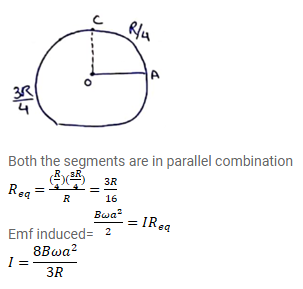# Consider the situation shown in the figure of the previous problem.

Question:

Consider the situation shown in the figure of the previous problem. Suppose the wire connecting 0 and $C$ has zero resistance but the circular loop has the resistance $\mathrm{R}$ uniformly distributed along its length. The rod $\mathrm{OA}$ is made to rotate with a uniform angular speed $\omega$ as shown in the figure. Find the current in the rod when $\angle \mathrm{AOC}=90^{\circ}$.

Solution: